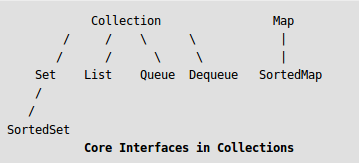# List Interface in Java with Examples

The Java.util.List is a child interface of Collection. It is an ordered collection of objects in which duplicate values can be stored. Since List preserves the insertion order, it allows positional access and insertion of elements. List Interface is implemented by the classes of ArrayList, LinkedList, Vector and Stack.Declaration:

`public abstract interface List extends Collection`

Creating List Objects:
List is an interface, and the instances of List can be created by implementing various classes in the following ways:

```List a = new ArrayList();
List c = new Vector();
List d = new Stack();
```

Generic List Object:
After the introduction of Generics in Java 1.5, it is possible to restrict the type of object that can be stored in the List. The type-safe List can be defined in the following way:

```// Obj is the type of object to be stored in List
List<Obj> list = new ArrayList<Obj> ();
```

Operations on List:
List Interface extends Collection, hence it supports all the operations of Collection Interface, along with following additional operations:

1. Positional Access:
List allows add, remove, get and set operations based on numerical positions of elements in List. List provides following methods for these operations:

• void add(int index, Object O): This method adds given element at specified index.
• boolean addAll(int index, Collection c): This method adds all elements from specified collection to list. First element gets inserted at given index. If there is already an element at that position, that element and other subsequent elements(if any) are shifted to the right by increasing their index.
• Object remove(int index): This method removes an element from the specified index. It shifts subsequent elements(if any) to left and decreases their indexes by 1.
• Object get(int index): This method returns element at the specified index.
• Object set(int index, Object new): This method replaces element at given index with new element. This function returns the element which was just replaced by new element.

 `// Java program to demonstrate positional access ` `// operations on List interface ` `import` `java.util.*; ` ` `  `public` `class` `ListDemo { ` `    ``public` `static` `void` `main(String[] args) ` `    ``{ ` `        ``// Creating a list ` `        ``List l1 = ``new` `ArrayList(); ` `        ``l1.add(``0``, ``1``); ``// adds 1 at 0 index ` `        ``l1.add(``1``, ``2``); ``// adds 2 at 1 index ` `        ``System.out.println(l1); ``// [1, 2] ` ` `  `        ``// Creating another list ` `        ``List l2 = ``new` `ArrayList(); ` `        ``l2.add(``1``); ` `        ``l2.add(``2``); ` `        ``l2.add(``3``); ` ` `  `        ``// Will add list l2 from 1 index ` `        ``l1.addAll(``1``, l2); ` `        ``System.out.println(l1); ` ` `  `        ``// Removes element from index 1 ` `        ``l1.remove(``1``); ` `        ``System.out.println(l1); ``// [1, 2, 3, 2] ` ` `  `        ``// Prints element at index 3 ` `        ``System.out.println(l1.get(``3``)); ` ` `  `        ``// Replace 0th element with 5 ` `        ``l1.set(``0``, ``5``); ` `        ``System.out.println(l1); ` `    ``} ` `} `

Output:

```[1, 2]
[1, 1, 2, 3, 2]
[1, 2, 3, 2]
2
[5, 2, 3, 2]
```
2. Search:
List provides methods to search element and returns its numeric position. Following two methods are supported by List for this operation:

• int indexOf(Object o): This method returns first occurrence of given element or -1 if element is not present in list.
• int lastIndexOf(Object o): This method returns the last occurrence of given element or -1 if element is not present in list.

 `// Java program to demonstrate search ` `// operations on List interface ` ` `  `import` `java.util.*; ` ` `  `public` `class` `ListDemo { ` `    ``public` `static` `void` `main(String[] args) ` `    ``{ ` `        ``// Type safe array list, stores only string ` `        ``List l = ``new` `ArrayList(``5``); ` `        ``l.add(``"Geeks"``); ` `        ``l.add(``"for"``); ` `        ``l.add(``"Geeks"``); ` ` `  `        ``// Using indexOf() and lastIndexOf() ` `        ``System.out.println(``"first index of Geeks:"` `                           ``+ l.indexOf(``"Geeks"``)); ` `        ``System.out.println(``"last index of Geeks:"` `                           ``+ l.lastIndexOf(``"Geeks"``)); ` `        ``System.out.println(``"Index of element"` `                           ``+ ``" not present : "` `                           ``+ l.indexOf(``"Hello"``)); ` `    ``} ` `} `

Output:

```first index of Geeks:0
last index of Geeks:2
Index of element not present : -1
```
3. Iteration:
4. Range-view:
List Interface provides a method to get the List view of the portion of given List between two indices. Following is the method supported by List for range view operation.

 `// Java program to demonstrate subList operation ` `// on List interface. ` `import` `java.util.*; ` `public` `class` `ListDemo { ` `    ``public` `static` `void` `main(String[] args) ` `    ``{ ` `        ``// Type safe array list, stores only string ` `        ``List l = ``new` `ArrayList(``5``); ` ` `  `        ``l.add(``"GeeksforGeeks"``); ` `        ``l.add(``"Practice"``); ` `        ``l.add(``"GeeksQuiz"``); ` `        ``l.add(``"IDE"``); ` `        ``l.add(``"Courses"``); ` ` `  `        ``List range = ``new` `ArrayList(); ` ` `  `        ``// Return List between 2nd(including) ` `        ``// and 4th element(excluding) ` `        ``range = l.subList(``2``, ``4``); ` ` `  `        ``System.out.println(range); ` `    ``} ` `} `

Output:

```[GeeksQuiz, IDE]
```

This article is contributed by Dharmesh Singh. If you like GeeksforGeeks and would like to contribute, you can also write an article using contribute.geeksforgeeks.org or mail your article to contribute@geeksforgeeks.org. See your article appearing on the GeeksforGeeks main page and help other Geeks.My Personal Notes arrow_drop_up

Improved By : Chinmoy Lenka

Article Tags :
Practice Tags :

22

Please write to us at contribute@geeksforgeeks.org to report any issue with the above content.